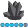## Graphing points with error bars

We don't yet have SAS/GRAPH and are considering purchasing.  Can you describe the process (short version) to add vertical error bars around a series of points?  These will be confidence intervals around the mean.  Would this be GPLOT or GCHART or other?  Anything else we should know? Thanks

2 REPLIES 2AncaTilea
Pyrite | Level 9

## Re: Graphing points with error bars

Hi.

Both will do it for you.

proc gchart data = sashelp.class;

vbar sex/ type=MEAN errorbar=BOTH clm=95 sumvar=age;

run; quit;

http://www.nesug.org/proceedings/nesug07/ff/ff17.pdf

For proc gplot you will have to calculate yourself the mean +/- s.e. to get the upper/lower Confidence Limits.Then use an overlay to plot.

42515 - Plot means with standard error bars from calculated data with PROC GPLOTDanH_sas
SAS Super FREQ

## Re: Graphing points with error bars

You can also use PROC SGPLOT. For bar charts, line charts, and dot plots, you can have the procedure compute three types of limits: clm, stderr, and stddev. YOu can also specify a multiplier for stderr and stddev.

proc sgplot data=sashelp.class;

vbar age / response=weight stat=mean limitstat=clm;

run;

For plots, you can use overlays to plot the limit bars:

proc sgplot data=somedata;

series x=xvar y=yvar;

scatter x=xvar y=yvar / yerrorupper=ulimitvar yerrorlower=llimitvar;

run;

The SG procedure were introduced in SAS 9.2 as part of SAS/GRAPH; however, as of SAS 9.3, they are part of BASE SAS.

Hope this helps!

Dan

Discussion stats
• 2 replies
• 2261 views
• 0 likes
• 3 in conversation